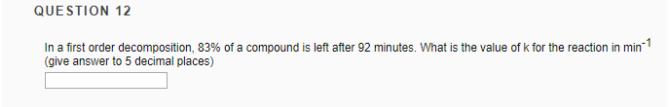# Problem: In a first order decomposition, 83% of a compound is left after 92 minutes. What is the value of k for the reaction in min-1 (give answer to 5 decimal places)

###### FREE Expert Solution
100% (114 ratings)###### Problem Details

In a first order decomposition, 83% of a compound is left after 92 minutes. What is the value of k for the reaction in min-1 (give answer to 5 decimal places)Frequently Asked Questions

What scientific concept do you need to know in order to solve this problem?

Our tutors have indicated that to solve this problem you will need to apply the Integrated Rate Law concept. You can view video lessons to learn Integrated Rate Law. Or if you need more Integrated Rate Law practice, you can also practice Integrated Rate Law practice problems.